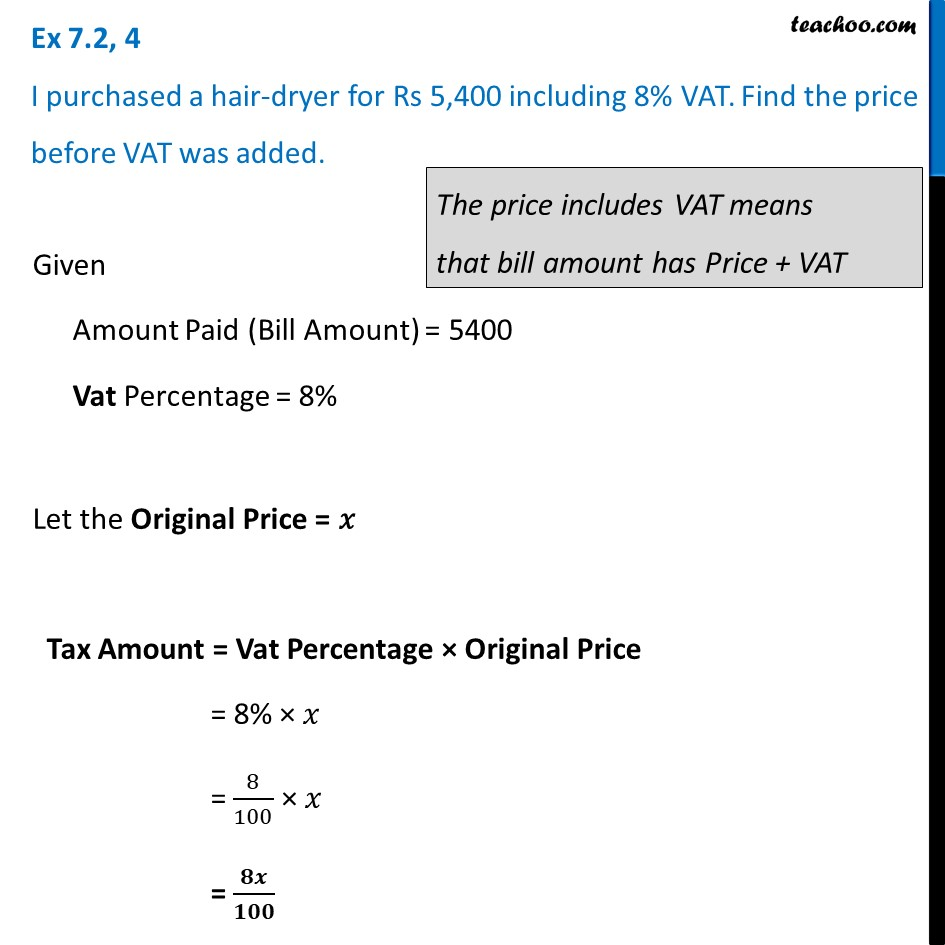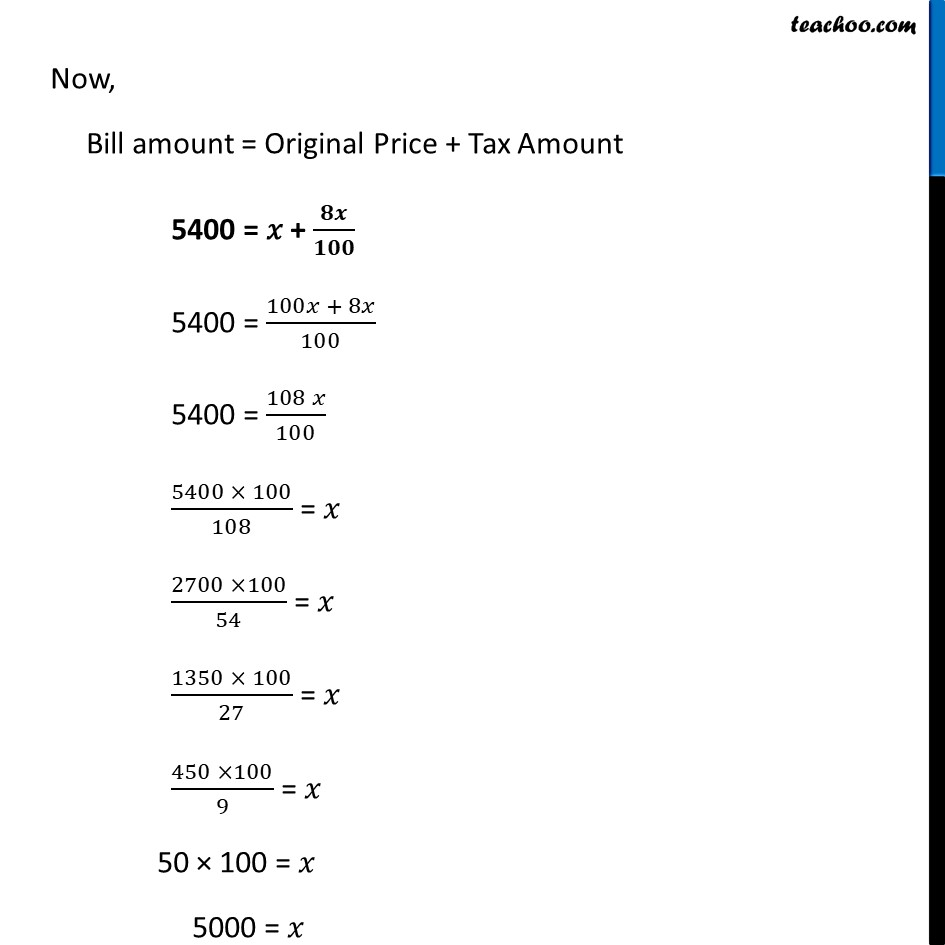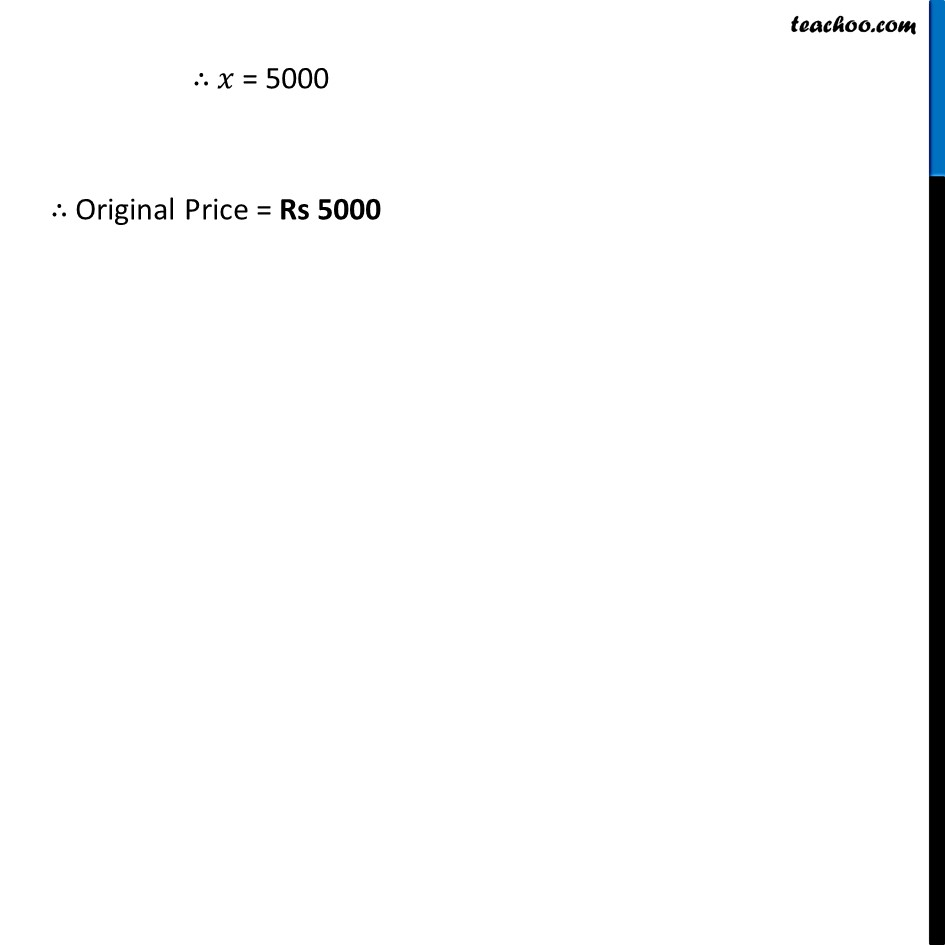Ex 7.2

Chapter 7 Class 8 Comparing Quantities
Serial order wiseLearn in your speed, with individual attention - Teachoo Maths 1-on-1 Class

### Transcript

Ex 7.2, 4 I purchased a hair-dryer for Rs 5,400 including 8% VAT. Find the price before VAT was added.Given Amount Paid (Bill Amount) = 5400 Vat Percentage = 8% Let the Original Price = 𝑥 Tax Amount = Vat Percentage × Original Price = 8% × 𝑥 = 8/100 × 𝑥 = 𝟖𝒙/𝟏𝟎𝟎 Now, Bill amount = Original Price + Tax Amount 5400 = 𝑥 + 𝟖𝒙/𝟏𝟎𝟎 5400 = (100𝑥 + 8𝑥)/100 5400 = (108 𝑥)/100 (5400 × 100)/108 = 𝑥 (2700 ×100)/54 = 𝑥 (1350 × 100)/27 = 𝑥 (450 ×100)/9 = 𝑥 50 × 100 = 𝑥 5000 = 𝑥 ∴ 𝑥 = 5000 ∴ Original Price = Rs 5000